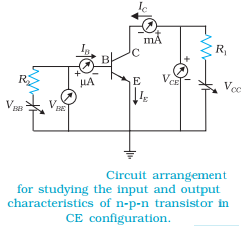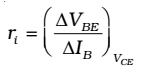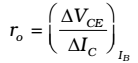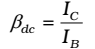# Transistor as an Amplifier

Amplification is the process of linearly increasing the amplitude of an electrical signal. A transistor can act as an amplifier directly using the gain, b. When a transistor is biased in the active (linear) region, the BE junction has a low resistance due to forward bias and the BC junction has a high resistance due to reverse bias.

## Common Emitter transistor characteristics:

When a transistor is used in CE configuration, the input is between the base and the emitter and the output is between the collector and the emitter. The variation of the base current IB with the base-emitter voltage VBE is called the input characteristic. Similarly, the variation of the collector current IC with the collector-emitter voltage VCE is called the output characteristic.Common Emitter transistor characteristics

The linear segments of both the input and output characteristics can be used to calculate some important ac parameters of transistors as shown below:

### Input resistance (ri):

This is defined as the ratio of change in base emitter voltage (∆VBE) to the resulting change in base current (∆IB) at constant collector-emitter voltage (VCE). This is dynamic (ac resistance) and as can be seen from the input characteristic, its value varies with
the operating current in the transistor:The value of ri can be anything from a few hundreds to a few thousand ohms.

### Output resistance (ro):

This is defined as the ratio of change in collector-emitter voltage (∆VCE) to the change in collector current (∆IC) at a constant base current IB.### Current amplification factor (β):

This is defined as the ratio of the change in collector current to the change in base current at a constant collector-emitter voltage (VCE) when the transistor is in active state.This is also known as small signal current gain and its value is very large.
If we simply find the ratio of IC and IB we get what is called dc β of the transistor. Hence,Since IC increases with IB almost linearly and IC= 0 when IB= 0, the values of both βdc and βac are nearly equal. So, for most calculations, βdc can be used. Both βac and βdc vary with VCE and IB (or IC) slightly.